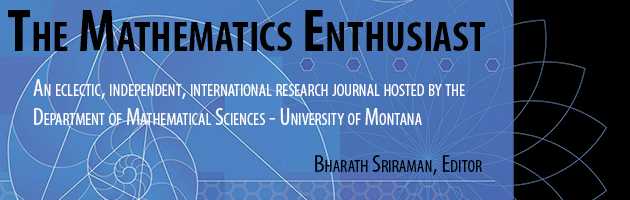•
•14

1-3

#### Abstract

System Dynamics (SD) modeling is a powerful analytical method used by professional scientists, academics, and governmental officials to study the behavior patterns of complex systems. Specifically through use of the Stella software, it is a method that I and others have used for over two decades with high school, and even middle school, math and science students. In this paper I describe an introduction to SD modeling intended for an algebra class (in either middle or high school). In the body of the paper, a nested sequence of simple bank account examples, increasing in complexity, is used to demonstrate a comparison between using a closed form approach and using Stella to mathematize each situation. The comparison, showing equivalent recursive equations, closed form equations, and Stella modeling diagrams, is designed to give the reader (algebra teacher, mathematics education decision-maker, researcher, or whomever) an accessible introduction to understanding Stella model diagrams and the mathematical engine operating under the “hood” of the software. In particular, I highlight the limitations of closed form equations to capture the needed problem elements beyond a certain level of complexity, even when the problem is still simple enough for analysis by quite young students using Stella. In the final section, I discuss how, once students become comfortable with the software, the level of sophistication of problems they can analyze (including complex problems) by designing and building Stella models is extensive, significantly beyond what they can analyze with equations. Then I point to limitations in the traditional math curriculum, manifest in the Common Core State Standards, in terms of failure to prepare students for modeling of complex dynamic systems, and the related failure to exploit the potential of new representational resources.

347

370

COinS